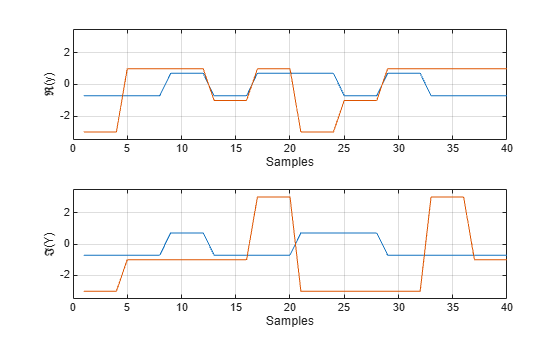# rectpulse

Rectangular pulse shaping

## Syntax

``Y = rectpulse(X,nsamp)``

## Description

example

````Y = rectpulse(X,nsamp)` performs rectangular pulse shaping on `X` and returns `Y`, increasing the sample rate of `X` by repeating each sample `nsamp` times.```

## Examples

collapse all

Create an array of modulated symbols. The first channel (column) contains QPSK-modulated symbols. The second channel contains 16QAM-modulated symbols.

```M_psk = 4; % PSK modulation order M_qam = 16; % QAM modulation order nsymb = 10; % Number of modulated symbols ch1 = pskmod(randi([0 M_psk - 1],nsymb,1),M_psk,pi/4); ch2 = qammod(randi([0 M_qam - 1],nsymb,1),M_qam); X = [ch1 ch2];```

Plot the real and imaginary parts of the modulated symbols.

```figure subplot(2,1,1) stem(real(X)) grid on xlabel('Symbols') ylabel('\Re(X)') subplot(2,1,2) stem(imag(X)) grid on xlabel('Symbols') ylabel('\Im(X)')```Set the number of samples per symbol.

`nsamp = 4;`

Perform rectangular pulse shaping and display the result.

```Y = rectpulse(X,nsamp); figure subplot(2,1,1) plot(real(Y),'-') grid on xlabel('Samples') ylabel('\Re(y)') ylim([-3.5 3.5]) subplot(2,1,2) plot(imag(Y),'-') grid on xlabel('Samples') ylabel('\Im(Y)') ylim([-3.5 3.5])```## Input Arguments

collapse all

Input symbols, specified as a vector or matrix. If `X` is a matrix with multiple rows, the function treats the columns as independent channels.

Data Types: `double` | `single`
Complex Number Support: Yes

Number of samples per symbol, specified as a positive integer.

Data Types: `double` | `single`

## Output Arguments

collapse all

Output samples, returned as a vector or matrix.

• If the input `X` is a vector, the output is an L-element vector consisting of each sample in `X` repeated `nsamp` times, where L is `length(X)*nsamp`.

• If the input `X` is a matrix, the function processes each column as an independent channel and the output is an L-by-M matrix, where L is `size(X,1)*nsamp` and M is `size(X,2)`.

The data type is the same as that of `X`.

## Version History

Introduced before R2006a